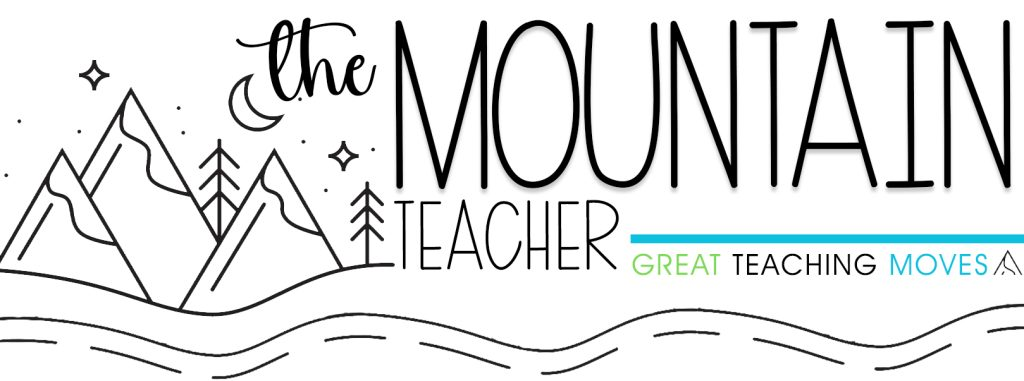# Multiply Making Groups of Objects Worksheets | Multiplication Activities

\$3.00

## Description

Need the perfect, ready-to-go multiplying by using groups of objects activities? Grab these multiplication activities for 2nd or 3rd grade students, including: making groups of objects to multiply lesson plan, warm up, turn & talk, instructional video, anchor charts, learning target, and practice worksheet.

This includes ONE multiplication activity that is a part of a bigger Multiplication Math Unit Bundle!

Multiply Groups of Objects Skills Covered:

• Students will look at groups of objects and come up with a multiplication equation to match
• Students will look at a multiplication equation and use groups of objects to solve the equation

Formats Included:

• Printable (color & black and white)
• TpT Easel

What’s Inside the Multiply Groups of Objects Activities

• Lesson Plan
• Learning Target
• Anchor Chart
• Instructional Video (covers the anchor charts, warm up and turn and talk)
• Warm Up Activity
• Turn & Talk Activity
• 1 Worksheet

How Long Will the Multiplication Activities Take?

These multiplying groups of objects activities for multiplication are designed to fill one lesson period. They will take anywhere from 25-90 minutes.

What standards are covered in the Multiplication Activities?

• 2.OA.C.4: Use addition to find the total number of objects arranged in rectangular arrays with up to 5 rows and up to 5 columns; write an equation to express the total as a sum of equal addends.

When to Use the Multiplication Activities:

• Math Block – Whole Group or Small Group
• Homeschool Environment
• Guest Teachers
• Substitute Teachers
• Student Teachers
• Centers
• Enrichment
• Remediation
• Special Education Environments

Need more multiplication lessons?

This lesson is part of a bigger unit – Multiplication Math Unit Bundle – which includes 15 days worth of lessons, assessments and more.

Get your plan time back and grab an entire year of math lessons!

## Reviews

There are no reviews yet.